# ISEE Middle Level Math : How to subtract variables

## Example Questions

### Example Question #11 : How To Subtract Variables

Simplify: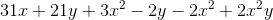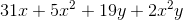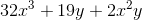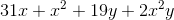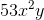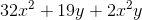Explanation:

Begin by putting similar variables together.  Remember that combinations of variables such as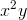are treated like a separate variable: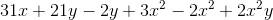Combine like terms: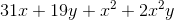You can then rearrange the variables to get the answer as written:### Example Question #4 : Expressions & Equations

Simplify: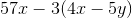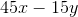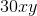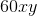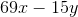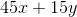Explanation:

Begin by distributing the: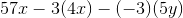Multiply each factor: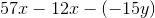Change the double negation to addition: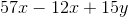Combine like terms:### Example Question #5 : Expressions & Equations

Simplify: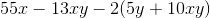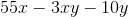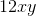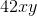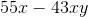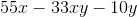Explanation:

Begin by distributing the: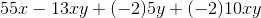Multiply all factors: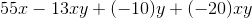Group together the only like factor (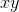):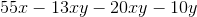Combine like terms:### Example Question #151 : Algebra

Simplify: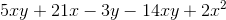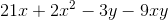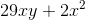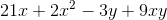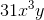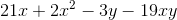Explanation:

Begin by moving all like terms next to each other.  Remember that you must treat every variable type separately: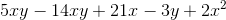Combine like terms: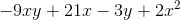You merely need to reorder the variables to get to the form in the answer choice.

### Example Question #161 : Ssat Middle Level Quantitative (Math)

Simplify: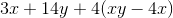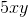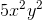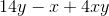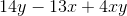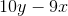Explanation:

Begin by distributing thethrough the group: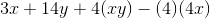Next, perform the multiplications: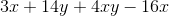Group the like terms: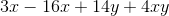Combine like terms: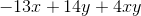Rearrange the terms to get the answer as it appears in the answer choices.

### Example Question #11 : How To Subtract Variables

Simplify: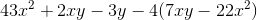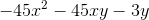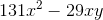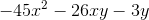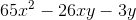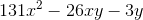Explanation:

Begin by multiplying through by: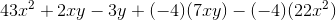Perform the multiplications: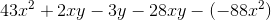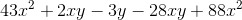Group like terms: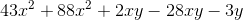Combine like terms: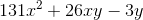### Example Question #161 : Algebra

Simplify: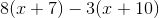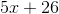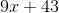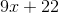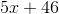Explanation: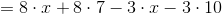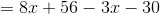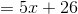### Example Question #163 : Ssat Middle Level Quantitative (Math)

Subtract in modulo 11: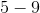Explanation: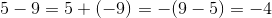in normal arithmetic.

In modulo 11 arithmetic, a negative number has 11 added to it as many times as necessary until a positive sum is reached: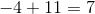Therefore,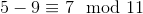### Example Question #164 : Ssat Middle Level Quantitative (Math)

Suppose you know the values of all variables in the expression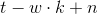and you want to evaluate the expression.

In which order will you carry out the operations?

Explanation:

By the order of operations, in the absence of grouping symbols, multiplication takes precedence over addition and subtraction. Addition and subtraction are then carried out with equal priority, but from left to right, so the subtraction is performed second and the addition last.

### Example Question #165 : Ssat Middle Level Quantitative (Math)

Which of the following phrases can be written as the algebraic expression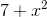?

Twice the sum of seven and a number

The square of the product of seven and a number

The square of the sum of seven and a number

Twice a number added to seven

The square of a number added to sevenisadded to seven;is the square of a number.is subsequently "the square of a number added to seven".